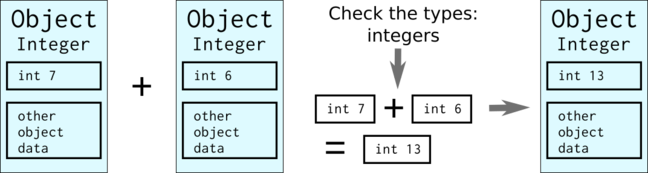## Want to keep learning?

This content is taken from the Partnership for Advanced Computing in Europe (PRACE)'s online course, Python in High Performance Computing. Join the course to learn more.
3.5

# Using static typing

Python is both a strongly typed and a dynamically typed language.

Strong typing means that variables do have a type and that the type matters when performing operations on a variable. Dynamic typing means that the type of the variable is determined only during runtime.

Due to strong typing, types need to be compatible with respect to the operand when performing operations. For example Python allows one to add an integer and a floating point number, but adding an integer to a string produces error.

Due to dynamic typing, in Python the same variable can have a different type at different times during the execution. Dynamic typing allows for flexibility in programming, but with a price in performance.

## Everything is an object

One of the key features of Python is that everything is an object, and the type is just one attribute of an object. As an illustration, we can assign a single integer to a variable, and use the Python built-in function dir for finding out the attributes of the object. If you execute the following two lines in an interactive interpreter, it should become clear that a Python integer is much more complex than just a number. Please feel free to experiment also with other types!

n = 5
dir(n)


The fact that everything is an object means that there is a lot of “unboxing” and “boxing” involved when Python performs operations with variables. For example, when just adding two integers

a = 7
b = 6
c = a + b


there are several steps Python needs to do:

1. Check the types of both operands
2. Check whether they both support the + operation
3. Extract the function that performs the + operation (due to operator overloading objects can have a custom definition for addition)
4. Extract the actual values of the objects
5. Perform the + operation
6. Construct a new integer object for the resultDue to the fact that Python is dynamically typed, the interpreter cannot know beforehand what type of objects one is dealing with, and everytime two variables are added one needs to perform all the above steps.

What if one knows that e.g. in a certain function the variables have always the same type? That’s where Cython steps in: Cython allows one to add static typing information so that boxing and unboxing are not needed, and one can operate directly with the actual values.

When Cythonizing a Python code, static type information can be added either:

• In function signatures by prefixing the formal arguments by their type
• By declaring variables with the cdef Cython keyword, followed by the the type

For example, a simple Python function adding two objects could be Cythonized as follows:

def add (int x, int y):
cdef int result
result = x + y
return result


The function works now only with integers but with less boxing/unboxing overheads.

The types provided in Cython code are C types, and the variables with type information are pure C variables and not Python objects. When calling a Cythonized function from Python, there is an automatic conversion from the Python object of actual arguments to the C value of formal argument, and when returning a C variable it is converted to corresponding Python object. Automatic conversions are carried out also in most cases within the Cython code where both Python objects and C variables are involved.

The table below lists the most common C types and their corresponding Python types. More information can be found in the Cython documentation.

From Python types To C types
int int, long
int, float float, double
str/bytes char *
From C types To Python types
int, long int
float, double float
char * str/bytes

## Static typing in Mandelbrot kernel

In week 1 we did a performance analysis of Mandelbrot fractal in Step 1.11. The analysis revealed that the kernel function in module mandelbrot.py was the most time critical one. Thus, let’s make mandelbrot.py into Cython module mandelbrot.pyx and introducing static typing to the function kernel.

Pure Python version was:

def kernel(zr, zi, cr, ci, lim, cutoff):
''' Computes the number of iterations n such that
|z_n| > lim, where z_n = z_{n-1}**2 + c.
'''
count = 0
while ((zr*zr + zi*zi) < (lim*lim)) and count < cutoff:
zr, zi = zr * zr - zi * zi + cr, 2 * zr * zi + ci
count += 1
return count


We can add type information both to the function signature and to the function body:

def kernel(double zr, double zi, double cr, double ci, double lim, int cutoff):
''' Computes the number of iterations n such that
|z_n| > lim, where z_n = z_{n-1}**2 + c.
'''
cdef int count = 0
while ((zr*zr + zi*zi) < (lim*lim)) and count < cutoff:
zr, zi = zr * zr - zi * zi + cr, 2 * zr * zi + ci
count += 1
return count


When comparing the performance of pure Python and Cythonized versions, we obtain the following results:

• Pure Python: 0.57 s
• Static type declarations in the kernel: 14 ms

Thus, we obtained a speed up of ~40 !